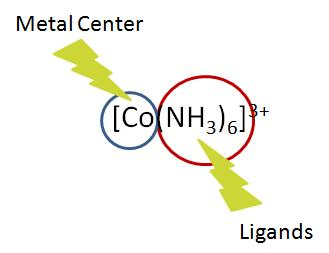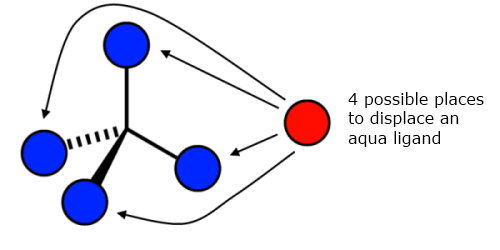# Complex-Ion Equilibria

When a metal ion reacts with a Lewis base in solution a complex ion is formed. This reaction can be described in terms of chemical equilibria.

## Introduction

A complex ion is comprised of two important parts: the central atom and its surrounding ligands. The central atom can be any metallic ion (usually a transition metal). The ligands are any combination of anions that can donate an electron pair, effectively meaning they are all Lewis bases. When combined they form coordinate covalent bonds.## Formation Constant

In general, chemical equilibrium is reached when the forward reaction rate is equal to the reverse reaction rate and can be described using an equilibrium constant, $$K$$. Complex ion equilibria are no exception to this and have their own unique equilibrium constant. This formation constant, $$K_f$$, describes the formation of a complex ion from its central ion and attached ligands. This constant may be called a stability constant or association constant; the units depend on the specific reaction it is describing. Common metal/ligand $$K_f$$ values are tabulated in Table E4.

At its most basic level, $$K_f$$ can be explained as the following, where $$M$$ is a metal ion, $$L$$ is a ligand, and $$x$$ and $$y$$ are coefficients:

$K_f = \dfrac{[M_xL_y]} {[M]^x[L]^y}$

$$K_f$$ values tend to be very large in magnitude because complex ion formation reactions heavily favor the product.

Example $$\PageIndex{1}$$

For example, observe the formation reaction of the dicyanoargentate(I) ion and the resulting formation constant expression:

$Ag^+_{(aq)} + 2CN^-\, _{(aq)} \rightleftharpoons Ag(CN)_2^- \, _{(aq)}$

$K_f = \dfrac{[Ag(CN)_2^-]}{[Ag^{+}][CN^{-}]^{2}} = 5.6 \times 10^{18}$

The larger the $$K_f$$ value of a complex ion, the more stable it is. Due to how large formation constants often are it is not uncommon to see them listed as logarithms in the form $$\log K_f$$. You may also see them in the form of a dissociation constant, $$K_d$$ (which should not be confused with pKa). This is merely the inverse value of the formation constant and as such describes the instability of a complex ion. It may help to think of $$K_f$$ and $$K_d$$ as stability and instability constants, respectively.

Using the above example, the dissociation constant expression would be:

$$K_f = \dfrac{1}{K_d}$$

$$K_{d} = \dfrac{1}{K_f} = \dfrac{[Ag^{+}][CN^{-}]^{2}}{[[Ag(CN)_2]^{-}]} = 1.8 \times 10^{-19}$$

In logarithm form $$K_f$$and $$K_d$$ would be:

$$\log K_{f} = \log(5.6 \times 10^{18}) = 18.7$$

$$\log K_{d} = \log(1.8 \times 10^{-19}) = -18.7$$

Example $$\PageIndex{2}$$: Stepwise Equilbria

One might assume that the formation of complex ions is a one step process, however this is not always the case. As an example let's look at the formation of tetraamminecuprate(II) ion in solution:

$[Cu(H_2O)_4]^{2+}_{(aq)} + 4NH_{3 \; (aq)} \rightleftharpoons [Cu(NH_3)_4]^{2+}_{(aq)} + 4H_2O_{(l)}$

The reason for using the hydrated form of the copper ion is that cations in solution usually exist in their hydrated form. It is because of this that the creation of tetraamminecuprate(II) ion is a multi-step affair, the ammine ligands have to displace the existing aqua ligands. In calculations however, the concentration of water and the number of aqua ligands are effectively constant meaning that they can be safely ignored. Each step of this process has its own formation constant, and as with other chemical equilibria, the overall reaction constant is the product of these stepwise formation constants.

Step 1:

$[Cu(H_2O)_4]^{2+}_{(aq)} + NH_{3 \; (aq)} \rightleftharpoons [Cu(H_2O)_3(NH_3)]^{2+}_{(aq)} + H_2O_{(l)}$

$K_{1} = \dfrac{[[Cu(H_2O)_3(NH_3)]^{2+}]}{[[Cu(H_2O)_4]^{2+}][NH_3]} = 1.9 \times 10^4$

Step 2:

$[Cu(H_2O)_3(NH_3)]^{2+}_{(aq)} + NH_{3 \; (aq)} \rightleftharpoons [Cu(H_2O)_2(NH_3)_2]^{2+}_{(aq)} + H_2O_{(l)}$

$K_{2} = \dfrac{[[Cu(H_2O)_2(NH_3)_2]^{2+}]}{[[Cu(H_2O)_3(NH_3)]^{2+}][NH_3]} = 3.9 \times 10^3$

Step 3:

$[Cu(H_2O)_2(NH_3)_2]^{2+}_{(aq)} + NH_{3 \; (aq)} \rightleftharpoons [Cu(H_2O)(NH_3)_3]^{2+}_{(aq)} + H_2O_{(l)}$

$K_{3} = \dfrac{[[Cu(H_2O)(NH_3)_3]^{2+}]}{[[Cu(H_2O)_2(NH_3)_2]^{2+}][NH_3]} = 1.0 \times 10^3$

Step 4:

$[Cu(H_2O)(NH_3)_3]^{2+}_{(aq)} + NH_{3 \; (aq)} \rightleftharpoons [Cu(NH_3)_4]^{2+}_{(aq)} + H_2O_{(l)}$

$K_{4} = \dfrac{[[Cu(NH_3)_4]^{2+}]}{[[Cu(H_2O)(NH_3)_3]^{2+}][NH_3]} = 1.5 \times 10^2$

Total

$K_f = \beta_4 = K_{1} \times K_{2} \times K_{3} \times K_{4} = 1.1 \times 10^{13}$

$K_f = \dfrac{[[Cu(H_2O)_3(NH_3)]^{2+}]}{[[Cu(H_2O)_4]^{2+}][NH_3]} \times \dfrac{[[Cu(H_2O)_2(NH_3)_2]^{2+}]}{[[Cu(H_2O)_3(NH_3)]^{2+}][NH_3]} \times \\ \dfrac{[[Cu(H_2O)(NH_3)_3]^{2+}]}{[[Cu(H_2O)_2(NH_3)_2]^{2+}][NH_3]} \times \dfrac{[[Cu(NH_3)_4]^{2+}]}{[[Cu(H_2O)(NH_3)_3]^{2+}][NH_3]}$

$= \dfrac{[[Cu(NH_3)_4]^{2+}]}{[[Cu(H_2O)_4]^{2+}][NH_3]^{4}} = K_f$

If you wanted to find the formation constant of one of the intermediate steps, you would simply take the product of the $$K$$ values up until that point. For example the formation constant of $$[Cu(H_2O)_2(NH_3)_2]^{2+}$$ would be $$\beta_2 = K_{1} \times K_{2} = 7.4 \times 10^7$$. As seen here and above the standard notation for these values is a lowercase beta.

You may notice that each stepwise formation constant is smaller than the one before it. This decreasing trend is due to the effects of entropy, causing each step to be progressively less likely to occur. You can think of this in the following way, continuing with the previous example:When the first ammine ligand goes to displace an aqua ligand it has four sites from which to choose from, making it "easier" to displace one. Yet with every step the number of sites decreases making it increasingly more difficult. As always though, there are exceptions to this rule. If the values do not continually decrease then the structure of the complex ion likely changed during one of the steps.

## Chelation Effect

Generally, complex ions with polydentate ligands have much higher formation constants than those with monodentate ligands. This additional stability is known as the chelation effect. For example, the formation of the bis(ethylenediamine)cuprate(II) ion is identical to the tetraamminecuprate(II) ion above except the ethylenediamine ligands displace two aqua ligands at a time. Observe the differences in formation constant (Table E4):

 Complex Ion Kf $$[Cu(NH_3)_4]^{2+}$$ $$1.1 \times 10^{13}$$ $$[Cu(en)_2]^{2+}$$ $$1.0 \times 10^{20}$$

## Solubility Effects

The formation of complex ions can sometimes explain the solubility of certain compounds in solution. For example, let's say we have a saturated solution of silver bromide ($$AgBr$$) which also contains additional undissolved solid silver bromide. If high enough concentrations of ammonia are added to the solution, the solid silver bromide will dissolve despite the solution previously having been saturated. This is because of the formation diammineargentate(I) ion, illustrated by the following chemical equation:

$$AgBr_{(s)} + 2NH_{3 \; (aq)} \rightarrow [Ag(NH_3)_2]^{+}_{(aq)} + Br^-_{(l)}$$

## References

1. Beck, Mihály T. Chemistry of Complex Equilibria. Revised ed. New York: Halsted Press, 1990.
2. Brown, Theodore, Eugene Lemay, Bruce Bersten, and Catherine Murphey. Chemistry: The Central Science. 10th ed. Andalusia, Alabama: Pearson Publications Company, 2006.
3. Petrucci, Harwood, Herring, and Madura. General Chemistry: Principles and Modern Applications. 9th ed. Upper Saddle River, New Jersey: Pearson Education, 2007.
4. Wilkinson, Geoffrey, Robert D. Gillard, and Jon A. McCleverty, eds. Comprehensive Coordination Chemistry: The Synthesis, Reactions, Properties & Applications of Coordination Compounds. First ed. Vol. 1. Oxford, England: Pergamon Press, 1987. N. pag. 7 vols.